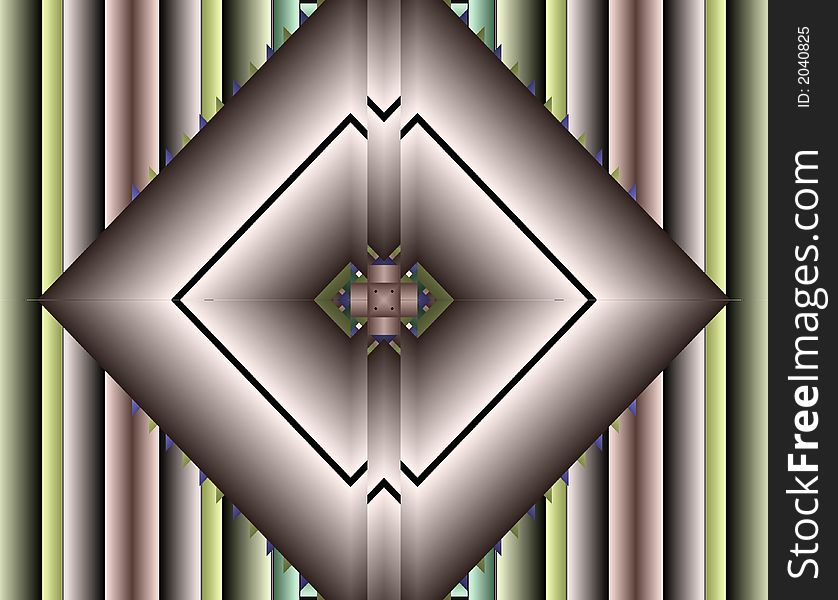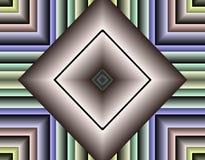Stock Image Of Fractal Geometry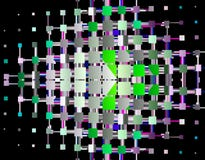Stock Image Of Fractal Geometry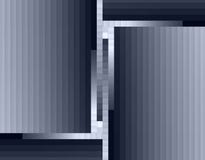Stock Image Of Fractal Geometry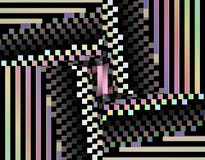Stock Image Of Fractal Geometry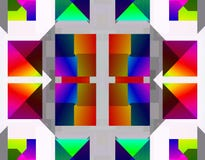Stock Image Of Fractal Geometry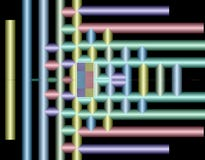Stock Image Of Fractal Geometry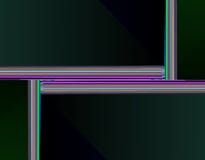Stock Image Of Fractal Geometry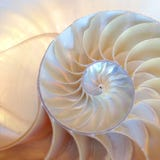Nautilus Shell Symmetry Fibonacci Half Cross Section Spiral Golden Ratio Structure Growth Close Up Back Lit Mother Of Pearl Close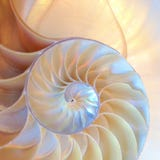Nautilus Shell Symmetry Fibonacci Half Cross Section Spiral Golden Ratio Structure Growth Close Up Back Lit Mother Of Pearl Close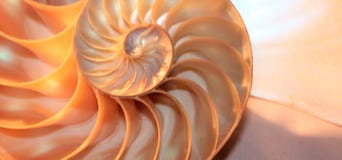Nautilus Shell Fibonacci Symmetry Cross Section Spiral Structure Growth Golden Ratio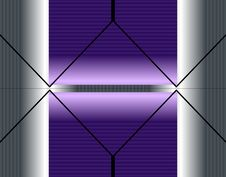Stock Image Of Fractal Geometry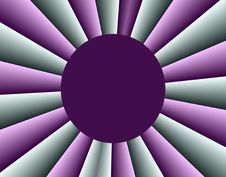Stock Image Of Fractal Geometry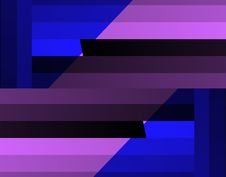Stock Image Of Fractal Geometry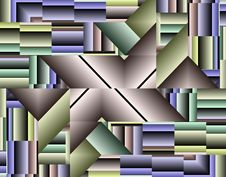Stock Image Of Fractal GeometryStock Image Of Fractal GeometryStock Image Of Fractal Geometry